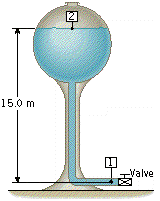Bernoulli's Equation of water tower

Homework Statement

The water tower in the drawing is drained by a pipe that extends to the ground. The flow is nonviscous.(b) What is the absolute pressure at point 1 when the valve is opened and the water is flowing? Assume that the water speed at point 2 is negligible.

(c) Assuming the effective cross-sectional area of the valve opening is 2.09 multiplied by 10-2 m2, find the volume flow rate at point 1.

The Attempt at a Solution

For part b:

p1 +density(g)(y) + .5(density)(v)^2 = p2 +density(g)(y) + .5(density)(v)^2
p1 + 0 + .5(1000)v^2 = 101000 + 1000(9.8)(15)

I have two variables p1 and v, so how can I solve this?